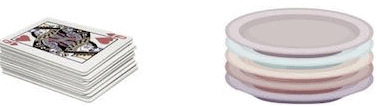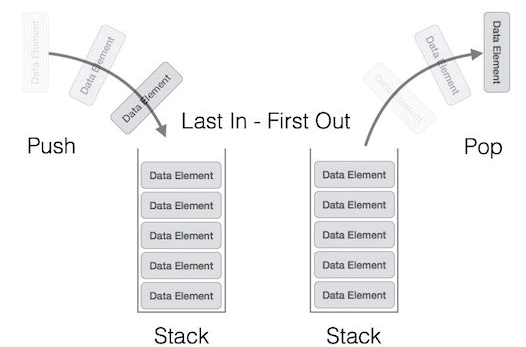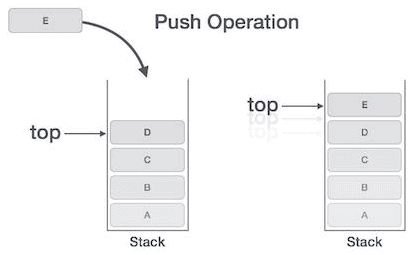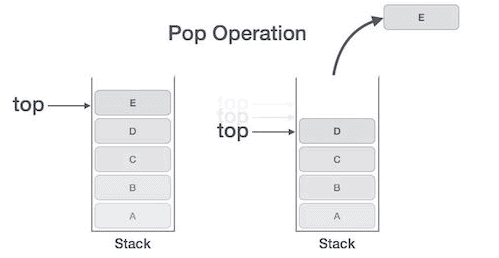# Stacks Notes | Study GATE Computer Science Engineering(CSE) 2023 Mock Test Series - GATE

## GATE: Stacks Notes | Study GATE Computer Science Engineering(CSE) 2023 Mock Test Series - GATE

The document Stacks Notes | Study GATE Computer Science Engineering(CSE) 2023 Mock Test Series - GATE is a part of the GATE Course GATE Computer Science Engineering(CSE) 2023 Mock Test Series.
All you need of GATE at this link: GATE

A stack is an Abstract Data Type (ADT), commonly used in most programming languages. It is named stack as it behaves like a real-world stack, for example – a deck of cards or a pile of plates, etc.A real-world stack allows operations at one end only. For example, we can place or remove a card or plate from the top of the stack only. Likewise, Stack ADT allows all data operations at one end only. At any given time, we can only access the top element of a stack.

This feature makes it LIFO data structure. LIFO stands for Last-in-first-out. Here, the element which is placed (inserted or added) last, is accessed first. In stack terminology, insertion operation is called PUSH operation and removal operation is called POP operation.

## Stack Representation

The following diagram depicts a stack and its operations −A stack can be implemented by means of Array, Structure, Pointer, and Linked List. Stack can either be a fixed size one or it may have a sense of dynamic resizing. Here, we are going to implement stack using arrays, which makes it a fixed size stack implementation.

## Basic Operations

Stack operations may involve initializing the stack, using it and then de-initializing it. Apart from these basic stuffs, a stack is used for the following two primary operations −

• push() − Pushing (storing) an element on the stack.

• pop() − Removing (accessing) an element from the stack.

When data is PUSHed onto stack.

To use a stack efficiently, we need to check the status of stack as well. For the same purpose, the following functionality is added to stacks −

• peek() − get the top data element of the stack, without removing it.

• isFull() − check if stack is full.

• isEmpty() − check if stack is empty.

At all times, we maintain a pointer to the last PUSHed data on the stack. As this pointer always represents the top of the stack, hence named top. The top pointer provides top value of the stack without actually removing it.

First we should learn about procedures to support stack functions −

### peek()

Algorithm of peek() function −

`begin procedure peek   return stack[top]   end procedure`

Implementation of peek() function in C programming language −

Example

`int peek() {   return stack[top];}`

### isfull()

Algorithm of isfull() function −

`begin procedure isfull   if top equals to MAXSIZE      return true   else      return false   endif   end procedure`

Implementation of isfull() function in C programming language −

Example

`bool isfull() {   if(top == MAXSIZE)      return true;   else      return false;}`

### isempty()

Algorithm of isempty() function −

`begin procedure isempty   if top less than 1      return true   else      return false   endif   end procedure`

Implementation of isempty() function in C programming language is slightly different. We initialize top at -1, as the index in array starts from 0. So we check if the top is below zero or -1 to determine if the stack is empty. Here's the code −

Example

`bool isempty() {   if(top == -1)      return true;   else      return false;}`

## Push Operation

The process of putting a new data element onto stack is known as a Push Operation. Push operation involves a series of steps −

• Step 1 − Checks if the stack is full.

• Step 2 − If the stack is full, produces an error and exit.

• Step 3 − If the stack is not full, increments top to point next empty space.

• Step 4 − Adds data element to the stack location, where top is pointing.

• Step 5 − Returns success.If the linked list is used to implement the stack, then in step 3, we need to allocate space dynamically.

### Algorithm for PUSH Operation

A simple algorithm for Push operation can be derived as follows −

`begin procedure push: stack, data   if stack is full      return null   endif      top ← top + 1      stack[top] ← dataend procedure`

Implementation of this algorithm in C, is very easy. See the following code −

Example

`void push(int data) {   if(!isFull()) {      top = top + 1;         stack[top] = data;   } else {      printf("Could not insert data, Stack is full.");   }}`

## Pop Operation

Accessing the content while removing it from the stack, is known as a Pop Operation. In an array implementation of pop() operation, the data element is not actually removed, instead top is decremented to a lower position in the stack to point to the next value. But in linked-list implementation, pop() actually removes data element and deallocates memory space.

A Pop operation may involve the following steps −

• Step 1 − Checks if the stack is empty.

• Step 2 − If the stack is empty, produces an error and exit.

• Step 3 − If the stack is not empty, accesses the data element at which top is pointing.

• Step 4 − Decreases the value of top by 1.

• Step 5 − Returns success.### Algorithm for Pop Operation

A simple algorithm for Pop operation can be derived as follows −

`begin procedure pop: stack   if stack is empty      return null   endif      data ← stack[top]      top ← top - 1      return dataend procedure`

Implementation of this algorithm in C, is as follows −

Example

`int pop(int data) {   if(!isempty()) {      data = stack[top];      top = top - 1;         return data;   } else {      printf("Could not retrieve data, Stack is empty.");   }}`
The document Stacks Notes | Study GATE Computer Science Engineering(CSE) 2023 Mock Test Series - GATE is a part of the GATE Course GATE Computer Science Engineering(CSE) 2023 Mock Test Series.
All you need of GATE at this link: GATEUse Code STAYHOME200 and get INR 200 additional OFF

## GATE Computer Science Engineering(CSE) 2023 Mock Test Series

136 docs|165 tests

Track your progress, build streaks, highlight & save important lessons and more!

,

,

,

,

,

,

,

,

,

,

,

,

,

,

,

,

,

,

,

,

,

;